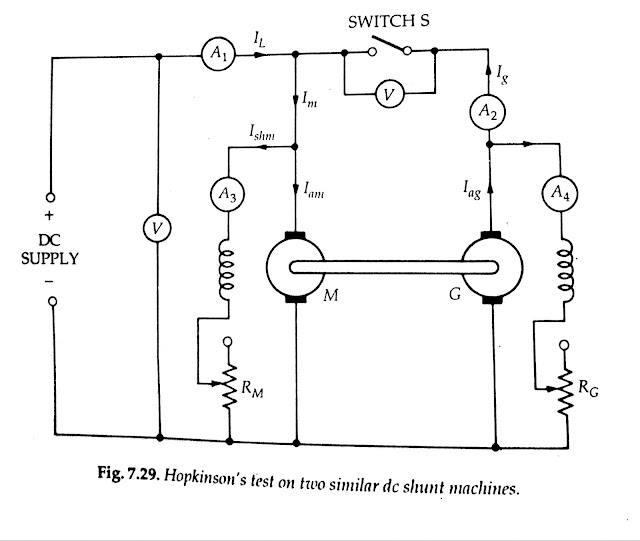# Hopkinson Test

Hopkinson Test requires two identical shunt machines which are coupled mechanically and also connected electrically in parallel. One of them acts as a motor and the other as the generator.

• The motor takes its input from the supply.

• The mechanical output of the motor drives the generator and the electrical output of the generator is used in supplying the input to the motor.

• Thus, the output of each machine is fed as input to the other.

• When both machines are run on full load, the input from the supply will be equal to the total losses of both the machines.

• Hence the power input from the supply is very small.

This test is also called

(a) regenerative test
(b) back-to-back test
(c) heat-run test.

## Hopkinson Test Circuit Diagram

[caption id="" align="alignnone" width="640"]Hopkinson test circuit diagram[/caption]

• The circuit diagram for the Hopkinson test is shown in Fig. 7.29 machine M is started from the supply as a motor with the help of a starter (not shown).

• The switch S is kept open.

• The field current of M is adjusted with the help of field rheostat RM to enable the motor to run at rated speed.

• Machine G acts as a generator. Since G is mechanically coupled to M, it runs at the rated speed of M.

• The excitation of the generator G is so adjusted with the help of its field rheostat RG that the voltage across the armature of G is slightly higher than the supply voltage.

• In actual practice, the terminal voltage of the generator is kept 1 or 2V higher than the supply busbar voltage.

• When this is achieved, that is, the voltage of the generator being equal and of the same polarity as the busbar voltage, the main switch S is closed and the generator is connected to the busbars.

• Thus, both the machines are now in parallel across the supply. Under this condition, the generator is said to float. That is, it is neither taking any current from nor giving any current to the supply.

• Any required load can now be thrown on the machines by adjusting the excitation of the machines with the help of field rheostats

## The efficiency of the Machine by Hopkinson Test

[caption id="" align="aligncenter" width="436"]The efficiency of the Machine by Hopkinson Test[/caption]

[ MCB ] Miniature Circuit Breakers

## The efficiency of the Generator And Motor

[caption id="" align="aligncenter" width="540"]The efficiency of the Generator And Motor[/caption]

1. The main advantages of using Hopkinson test for determination of efficiency.\

2. The total power taken from the supply is very low. Therefore this method is very economical.

3. The temperature rise and the commutation conditions can be checked under rated load conditions.

4. stray losses are considered, as both the machines are operated under rated load conditions.

5. Large machines can be tested at rated load without consuming much power from the supply.

6. Efficiency at different loads can be determined.